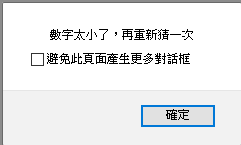#0

## 流程控制

1. 依撰寫程式的順序來執行。
2. 依條件的不同來做選擇
3. 重複執行直到答案為真的迴圈

### if 條件控制

``````var a = prompt("請輸入幸運數字","0");
if (a>=0) {
document.write("恭喜您很幸運");
}
``````

``````var a = prompt("請輸入幸運數字","0");
if (a>=0) {
document.write("恭喜您很幸運");
}
else {
document.write("不幸的開端");
}
``````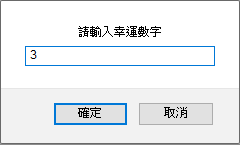### ?: 條件控制

``````var a = prompt("請輸入幸運數字","0");
(a >= 0) ? document.write("恭喜您很幸運"):
document.write("不幸的開端");
``````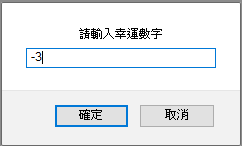### 多項選擇條件控制

``````var score = prompt("請輸入段考成績","0");

if (score <= 60 && score > 50) {
document.write("你獲得丁等，給我別混了");
}

else if (score <= 70 && score > 60) {
document.write("你獲得丙等，給我好好讀書!");
}

else if (score <= 80 && score > 70) {
document.write("你獲得乙等，還不錯，再努力");
}

else if (score <= 90 && score > 80) {
document.write("你獲得甲等，很厲害呢");
}

else if (score <= 100 && score > 90) {
document.write("你獲得優等，太優秀了!!");
}

else {
document.write("沒救了，本人不想評分");
}
``````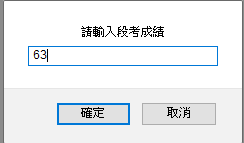### switch 多項條件判斷

``````var like = prompt("請選擇自己最喜歡的動漫? 1. Kanon 2. 月色真美 3. 天氣之子","1");

switch (like) {

case  "1":
document.write("我喜歡的動漫是 Kanon");
break;
case  "2":
document.write("我喜歡的動漫是 月色真美");
break;
case  "3":
document.write("我喜歡的動漫是 天氣之子");
break;
default:
document.write("請選擇正確的輸入數字");

}
``````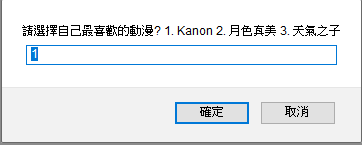### for 迴圈控制

for 迴圈控制的第一步是設定變數的初始設定，接下來設定這個變數需要執行幾次，最後是遞增與遞減的設定。

``````for (i=1; i<=9; i++) {
for (j=2; j<=9; j++) {
document.write(i +"*" + j + "=" + i*j + " ");
}

document.write("<br>" + "<br>");
}
``````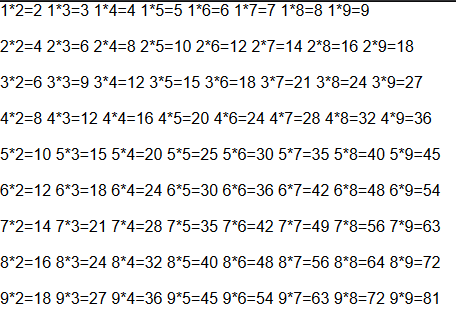### while 迴圈控制

while迴圈，第一步要先設定條件，當符合條件才會執行指定裡的程式，假如一直步符合條件的時候，才會跳出迴圈。

``````number = Math.floor(Math.random()*11); //隨機亂數設定
var guess = prompt("猜一個數字: ");
while (guess != number) {
if (number < guess) {
guess = prompt("數字太大了，再重新猜一次");
}
else {
guess = prompt("數字太小了，再重新猜一次");
}
}
``````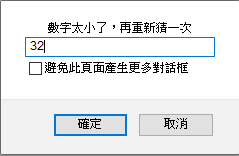### do while 迴圈控制

do while迴圈，與 while迴圈很類似，簡單來說就是先輸入值進行運算，最後才進行判斷的方法。

``````number = Math.floor(Math.random()*11);
var guess;
do {
guess = prompt("猜一個數字: ");
if (number < guess) {
}
else if (number > guess) {
}
} while (guess != number);
``````